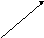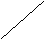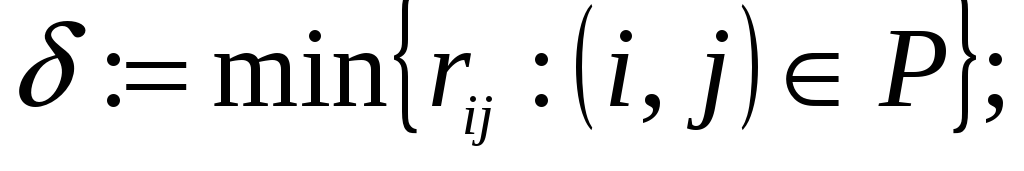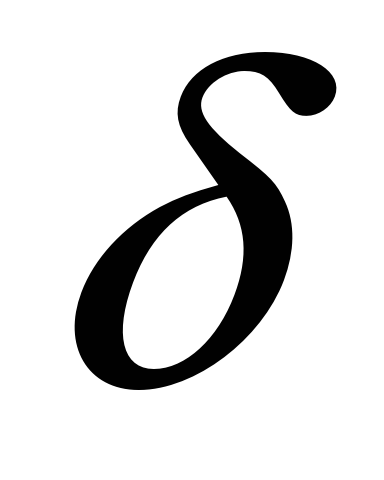# Maximum Flow Assumptions

 Property 6.2 For any flow x of value v in a network, the additional flow that can be sent from s to t is less than or equal to the residual capacity of any s - t cut. Generic Path Augmenting Algorithm Definition: Augmenting Path: directed path from s to t in the residual network Residual capacity of an augmenting path: minimum residual capacity of any arc in the augmenting path. 1 2s t 3 4 1 2s t 3 4 If the minimum residual capacity along an augmenting path is > 0 then we can push more flow along that path. We can build an algorithm that works by finding paths and pushing the maximum flow on them. algorithm augmenting path; begin x : = 0; while G(x) contains a directed path from node s to node t do begin identify an augmenting path P from node s to node t;augmentunits of flow along P and update G(x); end; end; Figure 6.12 Generic augmenting path algorithm. 1 2s t 3 4 1 2s t 3 4 1 2s t 3 4 1 2s t 3 4 Relationship between the original and residual networks We can keep track of xij or rij, because they have an equivalence. Augmenting path in original: Path P (need not be directed) from s to t such that xij < uij for forward arcs and xji > 0 for backward arcs. Can show equivalence to directed path in residual network. When we updated the flows we increased the xij values on the forward arcs and decreased the xji values on the backward arcs. If we use rij's how do we get xij's? Recall rij = uij - xij + xji or uij - rij = xij - xji if uij > rij let xij = uij - rij and xji = 0 if uij < rij let xij = 0 and xji = rij - uij Explanation of path augmenting when there are backward arcs. Labeling algorithm & maximum flow minimum cut theorem Discuss augmenting path algorithm in more detail. Specifically 1. How do we know if there are any more augmenting paths? 2. Will the algorithm terminate in an infinite number of steps? 3. Will the algorithm terminate at opt.? In general the algorithm fans out from s labeling nodes - we can label a node if we can reach that node in the residual network (that is there is a path with rij > 0 for all arcs in the path). When we label the sink, stop and augment flow. If we can't label the sink stop. algorithm labeling; begin label node t; while t is labeled do begin unlabel all nodes; set pred (j) : = 0 for each jN; label node s and set LIST : = {s}; while LIST   and t is unlabeled do begin remove a node i from LIST; for each arc (i, j) in the residual network emanating from node i do if rij > 0 and node j is unlabeled then set pred (j) : = i , label node j, and add j to LIST; end; if t is labeled then augment end; end; procedure augment; begin use the predecessor labels to trace back from the sink to the source to obtain an augmenting path P from node s to node t;augment  units of flow along P and update the residual capacities; Download 1 Mb.Share with your friends: